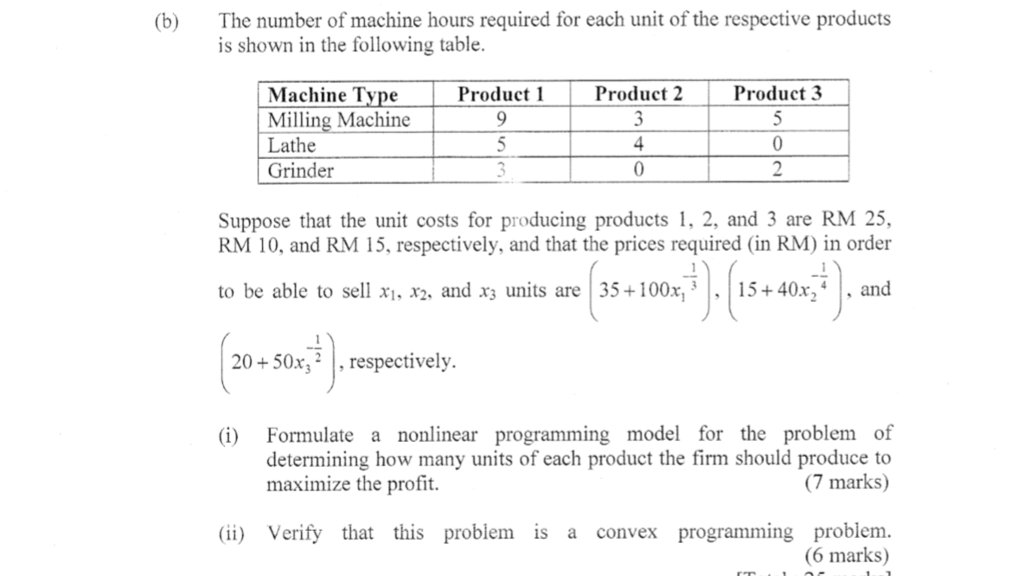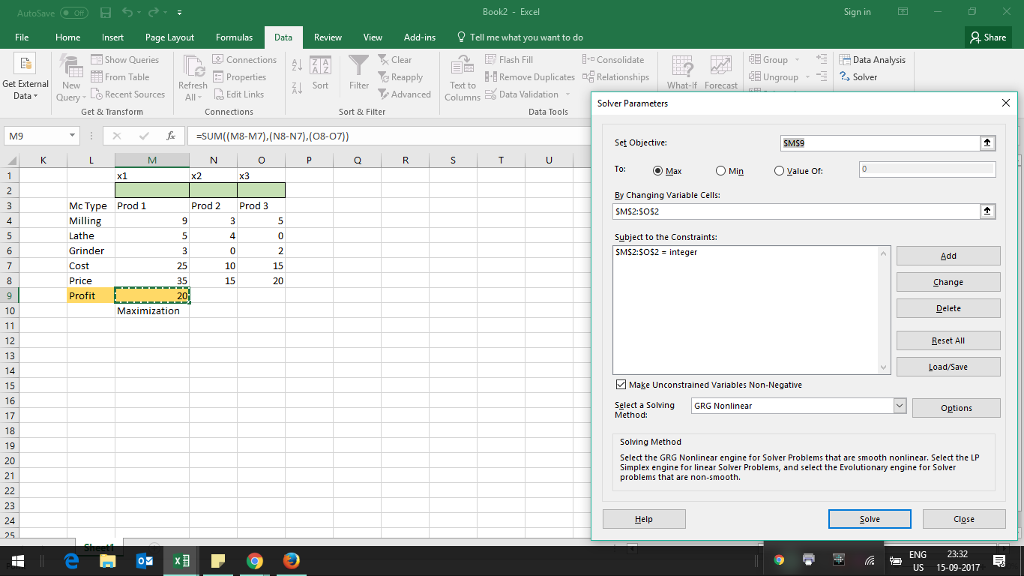# Question & Answer: (b) The number of machine hours required for each unit of the respective products…..(b) The number of machine hours required for each unit of the respective products is shown in the following table. Suppose that the unit costs for producing products 1, 2, and 3 are RM 25, RM 10, and RM 15, respectively, and that the prices required (in RM) in order to be able to sell x_1, x_2, and x_3 units are (35 + 100x^-1/3_1), (15 + 40x^-1/4_2), and (20 + 50x^-1/2_3), respectively. (i) Formulate a nonlinear programming model for the problem of determining how many units of each product the firm should produce to maximize the profit. (ii) Verify that this problem is a convex programming problem.